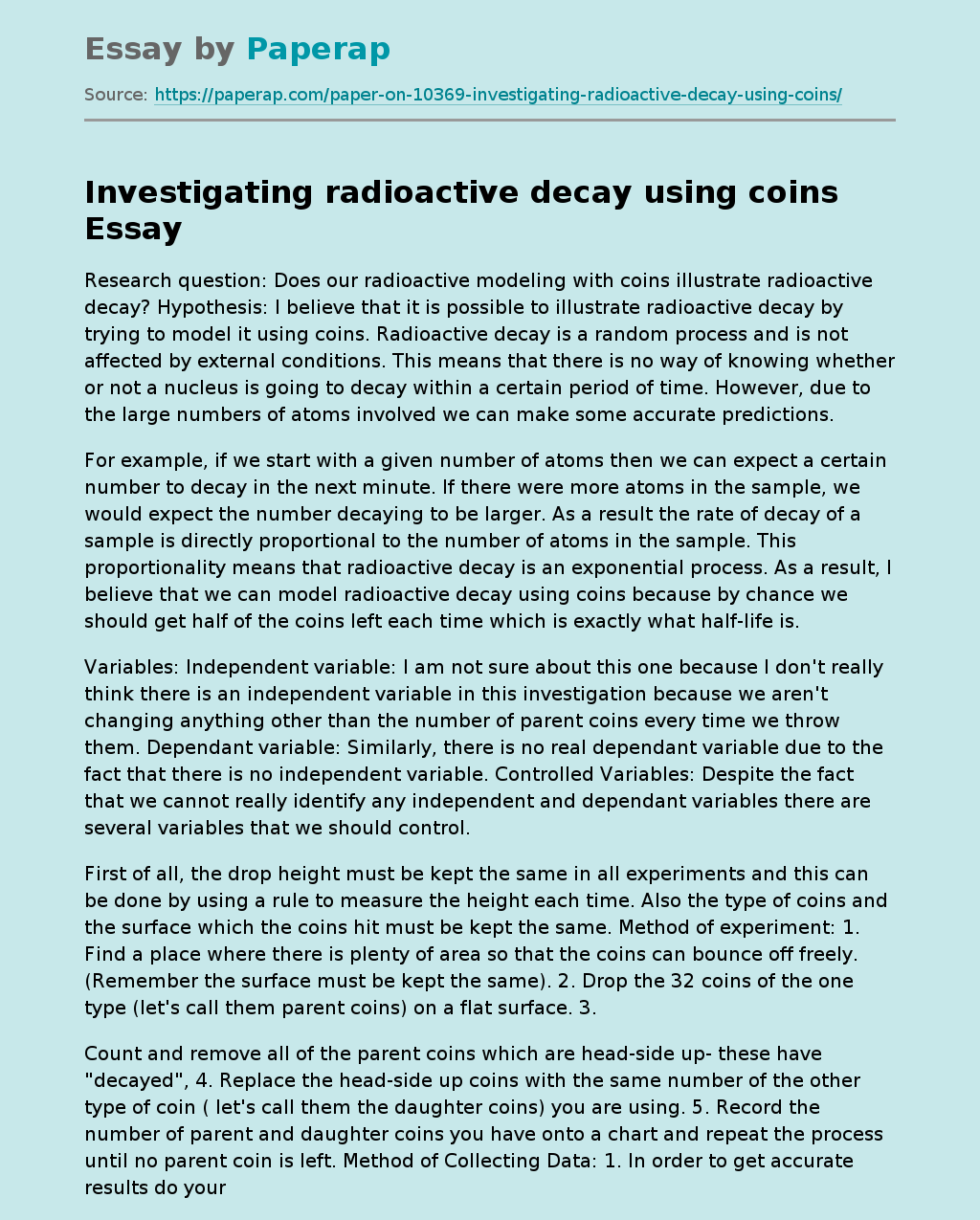I believe that it is possible to illustrate radioactive decay by trying to model it using coins. Radioactive decay is a random process and is not affected by external conditions. This means that there is no way of knowing whether or not a nucleus is going to decay within a certain period of time. However, due to the large numbers of atoms involved we can make some accurate predictions.

For example, if we start with a given number of atoms then we can expect a certain number to decay in the next minute.

If there were more atoms in the sample, we would expect the number decaying to be larger. As a result the rate of decay of a sample is directly proportional to the number of atoms in the sample. This proportionality means that radioactive decay is an exponential process. As a result, I believe that we can model radioactive decay using coins because by chance we should get half of the coins left each time which is exactly what half-life is.

Variables: Independent variable: I am not sure about this one because I don’t really think there is an independent variable in this investigation because we aren’t changing anything other than the number of parent coins every time we throw them. Dependant variable: Similarly, there is no real dependant variable due to the fact that there is no independent variable. Controlled Variables: Despite the fact that we cannot really identify any independent and dependant variables there are several variables that we should control.

Get quality help nowBella HamiltonVerified

Proficient in: Atom5 (234)

“ Very organized ,I enjoyed and Loved every bit of our professional interaction ”+84 relevant experts are online

First of all, the drop height must be kept the same in all experiments and this can be done by using a rule to measure the height each time. Also the type of coins and the surface which the coins hit must be kept the same. Method of experiment:

1. Find a place where there is plenty of area so that the coins can bounce off freely. (Remember the surface must be kept the same).
2. Drop the 32 coins of the one type (let’s call them parent coins) on a flat surface.
3. Count and remove all of the parent coins which are head-side up- these have “decayed”.
4. Replace the head-side up coins with the same number of the other type of coin ( let’s call them the daughter coins) you are using.
5. Record the number of parent and daughter coins you have onto a chart and repeat the process until no parent coin is left.

In order to get accurate results do your experiment about three times and then do an average. Data Collection and Processing Data collection: Now in order to answer my research question it is vital that we draw a graph because if the graph illustrates an exponential decay curve then it would support my theory that you can in fact model radioactive decay but if it doesn’t illustrate an exponential curve then it wouldn’t support my theory. Conclusion and Evaluation Conclusion: In essence, the results support my theory that it is possible to model radioactive decay using coins.

From the first graph we can clearly see that we have an exponential graph which is what radioactive decay is due to the fact that the rate of decay of a sample is directly proportional to the number of atoms in the sample. The other graph serves to illustrate that as the decay of the radioactive ‘parent’ nuclei occurs, there is an increase in the stable ‘daughter’ nuclei. Speaking about errors in this modeling exercise is very hard because this isn’t really an experiment. All we are really doing is throwing coins onto a flat surface and looking at the outcome i. e. the number of head-side up parent coins.

Systematic errors couldn’t have occurred because we aren’t using any instruments to measure anything. The only random error that could have occurred would be us missing one of the head-side up coins and not removing it when we had to. Evaluation: Again because this isn’t an experiment in which we have a definite independent variable and a dependant variable, there are no real weaknesses or limitations that occurred. The only weakness I can think of is that this modeling exercise relies a lot on chance.

In other words, it just might have been so that not approximately half of the coins were taken of each time. It would have been a total mess if for example the first few tries only 1 coin is head-side up and then after let’s say the 5th try all of them come up as head-side up. That would totally mess all the results and wouldn’t support the theory of radioactivity. Other than that I cannot think of any weaknesses. Improvements: Thinking of realistic improvement for this modeling exercise isn’t possible because it is a modeling exercise and not an experiment.

However, it never hurts to take more trial  perhaps 10 trials and then taking averages. This would give more accurate results but then again because this experiment relies too much on chance, doing 10 trials might make the results even worse. Another improvement can be is to use much more coins, for example a hundred coins. This gives a greater possibility to monitor radioactive decay. I am sorry that I couldn’t do all experiments but as you know we the chemists also had to do chemistry investigations and therefore we didn’t have enough time to do all the experiments.